# Medical Image Analysis with Deep Learning — II

In the last article we went through some basics of image-processing using OpenCV and basics of DICOM image. In this article we will talk about basics of deep learning from the lens of Convolutional Neural Nets. In the next article we will use Kaggle’s lung cancer data-set, review the key items to look for in a lung cancer DICOM image and use Kera’s to develop a model to predict lung cancer.

### Basic Convolutional Neural Nets (CNN)

In order to understand basics of CNN, we need to understand what is convolution.

What is convolution?

Wikipedia defines convolution as “a mathematical operation on two functions (f and g); it produces a third function, that is typically viewed as a modified version of one of the original functions, giving the integral of the point-wise multiplication of the two functions as a function of the amount that one of the original functions is translated.” The easy way to understand this by thinking of it as a sliding window function applied to a matrix.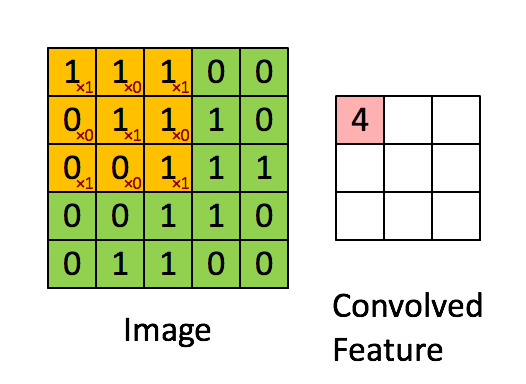Convolution with 3×3 Filter. Source: http://deeplearning.stanford.edu/wiki/index.php/Feature_extraction_using_convolution

The figure above shows the sliding window applied on the matrix in green, where sliding window matrix is in red. The output is the convolved Feature matrix. The figure below shows the convolution of two square pulses (blue and red) and the results.

Jeremy Howard, in his MOOC explains convolution using an excel sheet, which is a great way to understand the fundamentals. Consider 2 matrices f and g. The output of convolution of f and g, is the third matrix “Conv layer 1” given by the dot-product of of the 2 matrices. The dot product of 2 matrices is a scalar as shown below. An excellent source of math functions can be found here.

Lets use excel as Jeremy suggests, our input matrix is function f() and sliding window matrix is filter function g(). The dot product is the sum-product of the 2 matrices in excel as shown below.

Lets extend this to an image of alphabet “A”. As we know any image is made of pixels. So our input matrix f is “A”. We select our sliding window function to be a random matrix g. Then the convoluted output for the dot product of this matrix is shown below. Send me a note if you would like a copy of this excel sheet.

What are Convolutional Neural Nets (CNN) ?

In my point of view a simple Convolutional Neural Net (CNN) is a sequence of layers. Each layer has some specific functions. Each convolutional layer is 3 dimensional, so we use volume as the metric. Further, each layer of a CNN transforms one volume of activations to another through a differentiable function. Such a function is called activation or transfer function.

The different types of entities of CNN are : Input , Filters (or Kernels), Convolutional Layer, Activation Layer, Pooling Layer, and Batch Normalization layer. The combination of these layers in different permutations and of course some rules give us different deep learning architectures.

Input Layer : The usual input to a CNN is an n-dimensional array. For an image we have input with 3 dimensions — length, width and depth (which are the color channels)

Filters or Kernels : As shown in the figure from RiverTrail below, a filter or kernel slides to every position of the image and computes a new pixel as a weighted sum of the pixels it floats over. In our excel example above our filter is g, moves over the input matrix f.

Convolutional Layer: A layer of dot product of input matrix and kernel gives a new matrix know as the convolutional matrix or layer.

A very good visual chart understanding how padding, strides and transpose work can be found below.

Activation Layer :

Activation functions can be classified into 2 categories based — Saturated and Non-Saturated.

Saturated activation functions are sigmoid and tanh, whereas non-saturated are ReLU and its variants.The advantage of using non-saturated activation function lies in two aspects:

1. The first is to solve the so called “exploding/vanishing gradient”.
2. The second is to accelerate the convergence speed.

Sigmoid: takes a real-valued input and squashes it to range between [0,1]

σ(x) = 1 / (1 + exp(−x))

tanh: takes a real-valued input and squashes it to the range [-1, 1]

tanh(x) = 2σ(2x) − 1

ReLU

ReLU stands for Rectified Linear Unit. It is the max function(x,0) with input x e.g. matrix from a convolved image. ReLU then sets all negative values in the matrix x to zero and all other values are kept constant.ReLU is computed after the convolution and therefore a nonlinear activation function like tanh or sigmoid. This was first discussed by Geoff Hinton in his nature paper.

#### ELUs

Exponential linear units try to make the mean activations closer to zero which speeds up learning. ELUs also avoid a vanishing gradient via the identity for positive values. It has been shown that ELUs obtain higher classification accuracy than ReLUs. A very good detailed poster on ELU can be found here .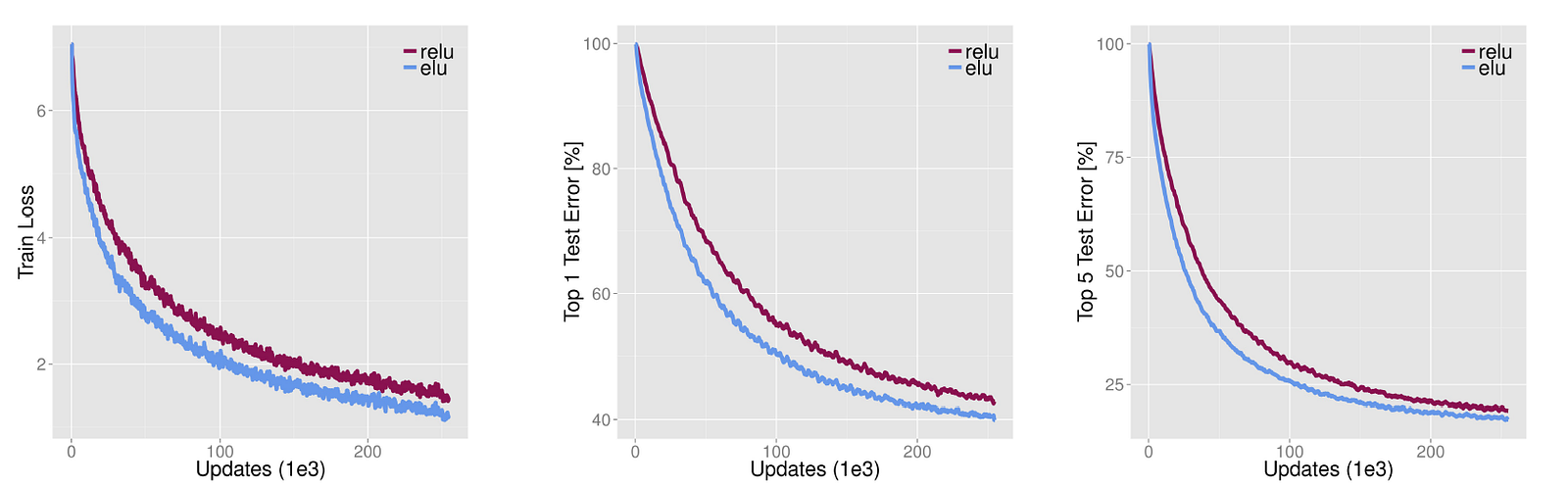Source: http://image-net.org/challenges/posters/JKU_EN_RGB_Schwarz_poster.pdf [15 layer CNN with stacks of (1×96×6, 3×512×3, 5×768×3, 3×1024×3, 2×4096×FC, 1×1000×FC) layers×units×receptive fields or fully-connected (FC). 2×2 max-pooling with a stride of 2 after each stack, spatial pyramid pooling with 3 levels before the first FC layer.]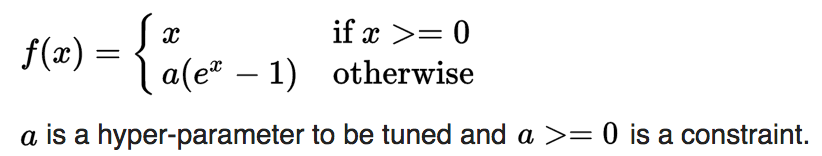Source : Wikipedia

#### Leaky ReLUs

In contrast to ReLU, in which the negative part is totally dropped, leaky ReLU assigns a non-zero slope to it. Leaky Rectified Linear activation is first introduced in acoustic model(Maas et al., 2013). Mathematically, we have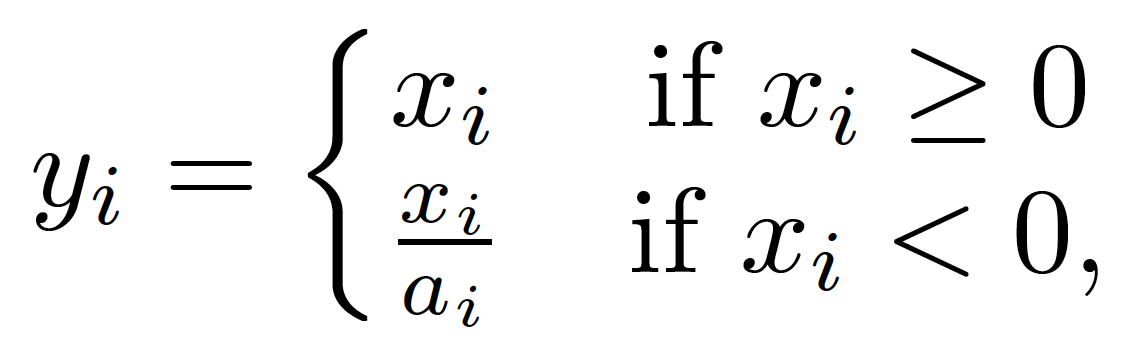Source: Empirical Evaluation of Rectified Activations in Convolution Network

where ai is the fixed parameter in the range (1,+infinity).

Parametric Rectified Linear Unit (PReLU)

PReLU can be considered as a variant of Leaky ReLU. In PReLU, the slopes of negative part are learned form data rather than predefined. The authors claimed that PReLU is the key factor of surpassing human-level performance on ImageNet classification (Russakovsky et al., 2015) task. It is the same as leaky ReLU with the exception that ai is learned in the training via back propagation.

Randomized Leaky Rectified Linear Unit (RReLU)

Randomized rectified linear unit (RReLU) are also a variant of Leaky ReLU. In RReLU, the slopes of negative parts are randomized in a given range in the training, and then fixed in the testing. The highlight of RReLU is that in training process, aji is a random number sampled from a uniform distribution U(l,u). Formally, we have:

A comparison between ReLU, Leaky ReLU, PReLU and RReLU is shown below.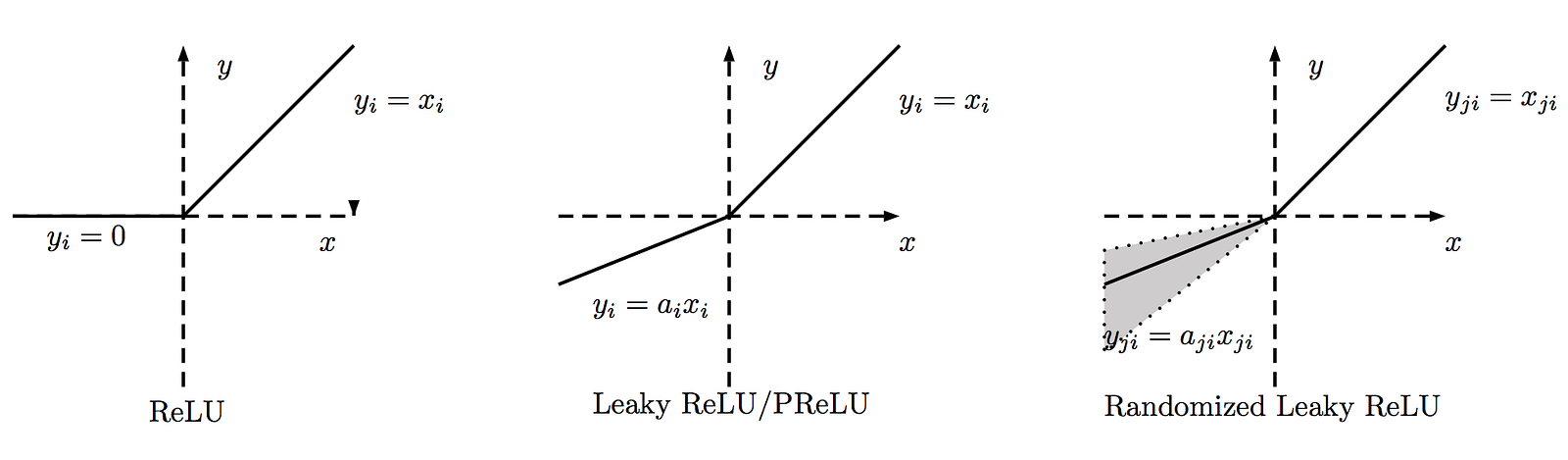Source :https://arxiv.org/pdf/1505.00853.pdf ReLU, Leaky ReLU, PReLU and RReLU. For PReLU, ai is learned and for Leaky ReLU ai is fixed. For RReLU, aji is a random variable keeps sampling in a given range, and remains fixed in testing.

Noisy Activation functions

These are activation functions, extended to include Gaussian noise. Good understanding on how Noise helps can be found here.

Pooling Layer:

The goal of a Pooling layer is to progressively reduce the spatial size of the matrix to reduce the amount of parameters and computation in the network, and hence to also control overfitting. The Pooling Layer operates independently on every depth slice of the input and resizes it spatially, using the MAX or Average operation. The most common form is a pooling layer with filters of size 2x2 applied with a stride of 2 downsamples every depth slice in the input by 2 along both width and height, discarding 75% of the activations. Every MAX operation would in this case be taking a max over 4 numbers (little 2x2 region in some depth slice). The depth dimension remains unchanged. More generally, the pooling layer:

Note: Here we slide our 2 x 2 window by 2 cells (also called ‘stride’) and take the maximum value in each region.

Batch Normalization layer:

Batch normalization is an effective way of normalizing each intermediate layer including the weights and activation functions. There are two main benefits for batchnorm:

1. Adding batchnorm to a model can result in 10x or more improvements in training speed
2. Because normalization greatly reduces the ability of a small number of outlying inputs to over-influence the training, it also tends to reduce overfitting.

Details about batch normalization can be found here or check Jeremy’s MOOC.

Fully Connected layer:

The Fully Connected layer is a traditional Multi Layer Perceptron that uses a softmax activation function in the output layer. The term “Fully Connected” implies that every neuron in the previous layer is connected to every neuron on the next layer. A softmax function is a generalization of the logistic function that “squashes” a K-dimensional vector, of arbitrary real values to a K-dimensional vector of real values in the range (0, 1) that add up to 1.